# RD Sharma Solutions For Class 7 Maths Chapter 16 Congruence Exercise 16.1

Exercise 16.1 of Chapter 16 covers important topics like congruent figures, congruence of line segments and various types of line segments along with illustrative examples to improve conceptual knowledge. Students who find it difficult in solving the exercise wise problems of RD Sharma textbook can access PDF of solutions. The problems are solved by our experts in the best possible way to make it easier for the students during exam preparation. For further reference, the students can download RD Sharma Solutions for Class 7 Maths Chapter 16 Congruence Exercise 16.1 PDF, from the links given here. Let us have a look at some of the important topics present in this exercise.

• Congruent figures
• Congruence of line segments
• Congruence of two angles
• Congruence of two squares
• Congruence of two circles

## Download the PDF of RD Sharma Solutions For Class 7 Maths Chapter 16 – Congruence Exercise 16.1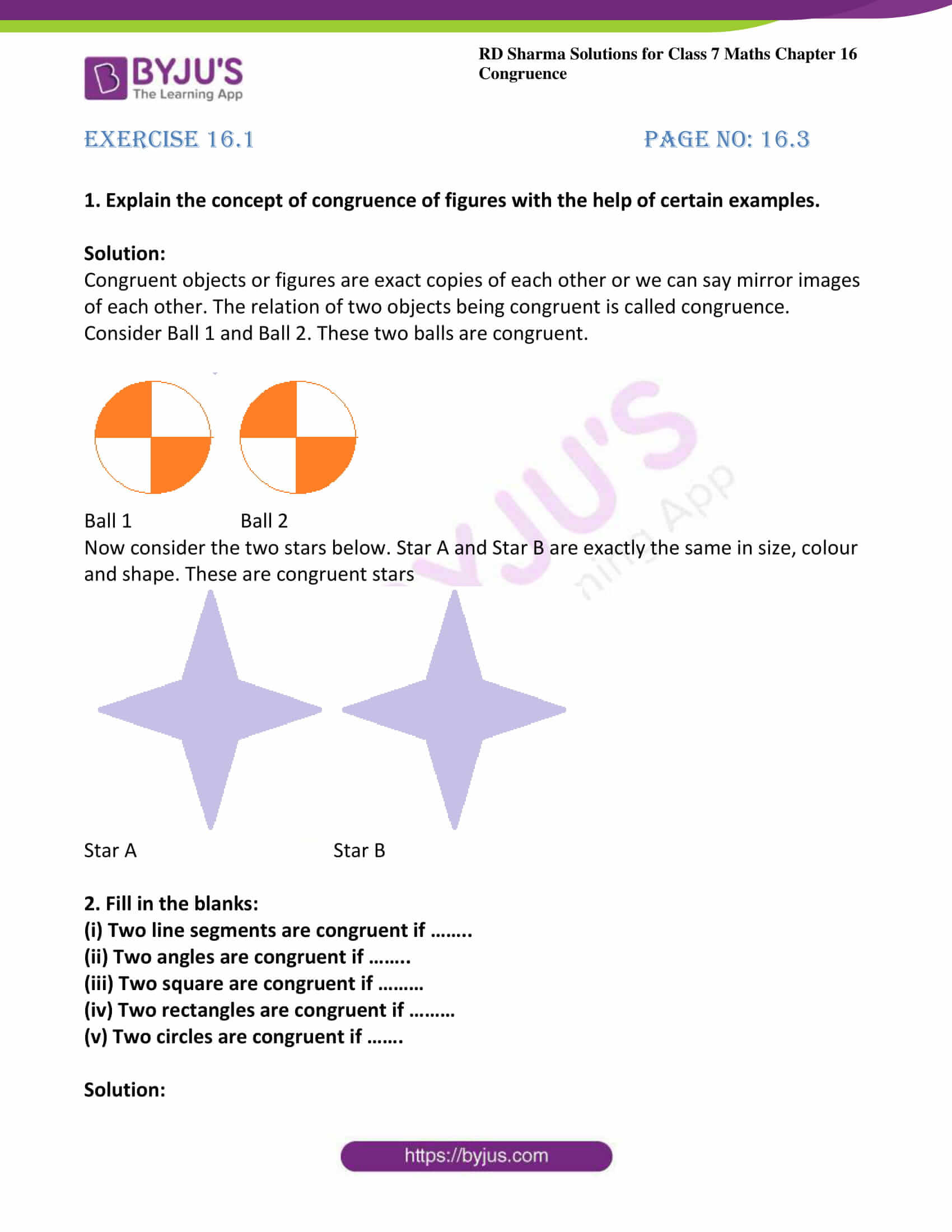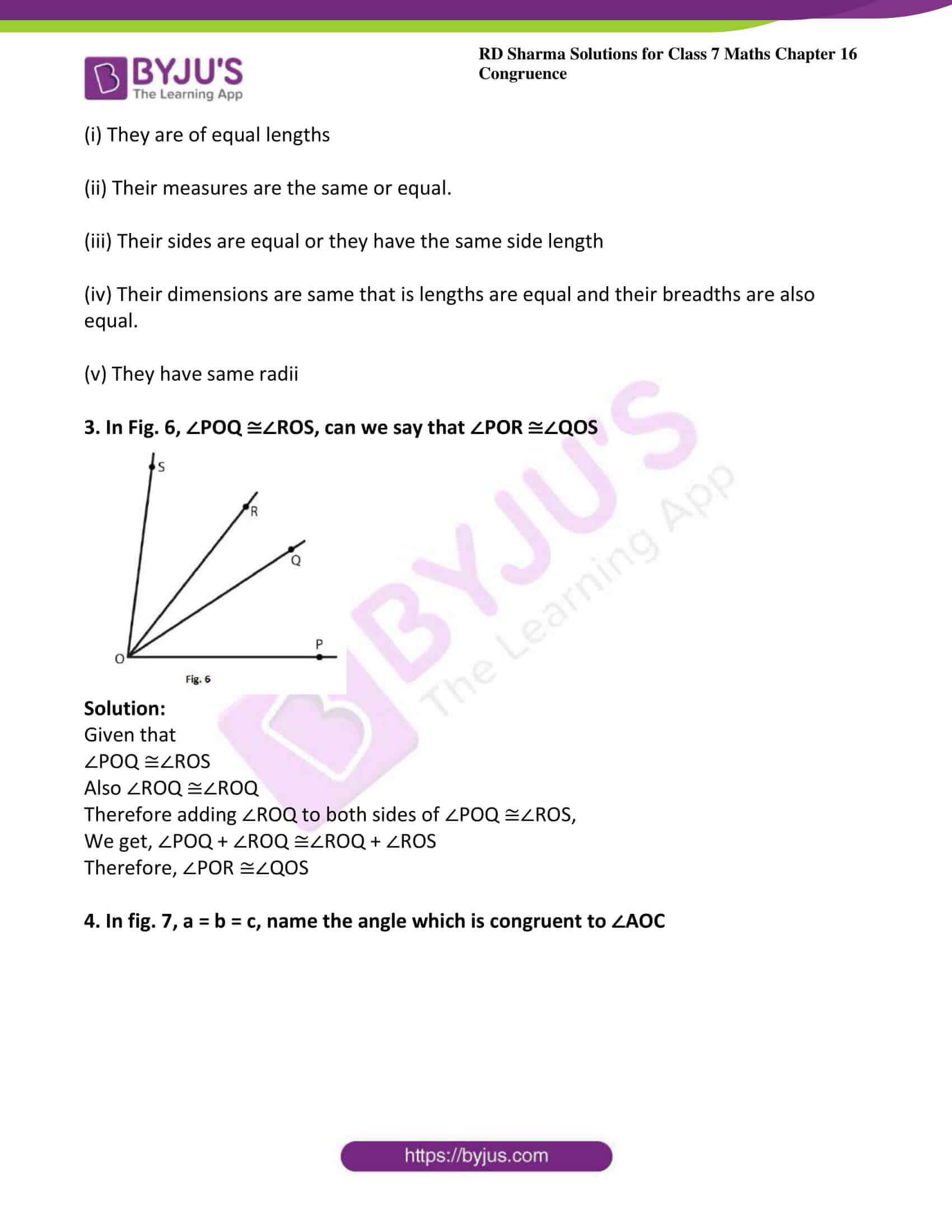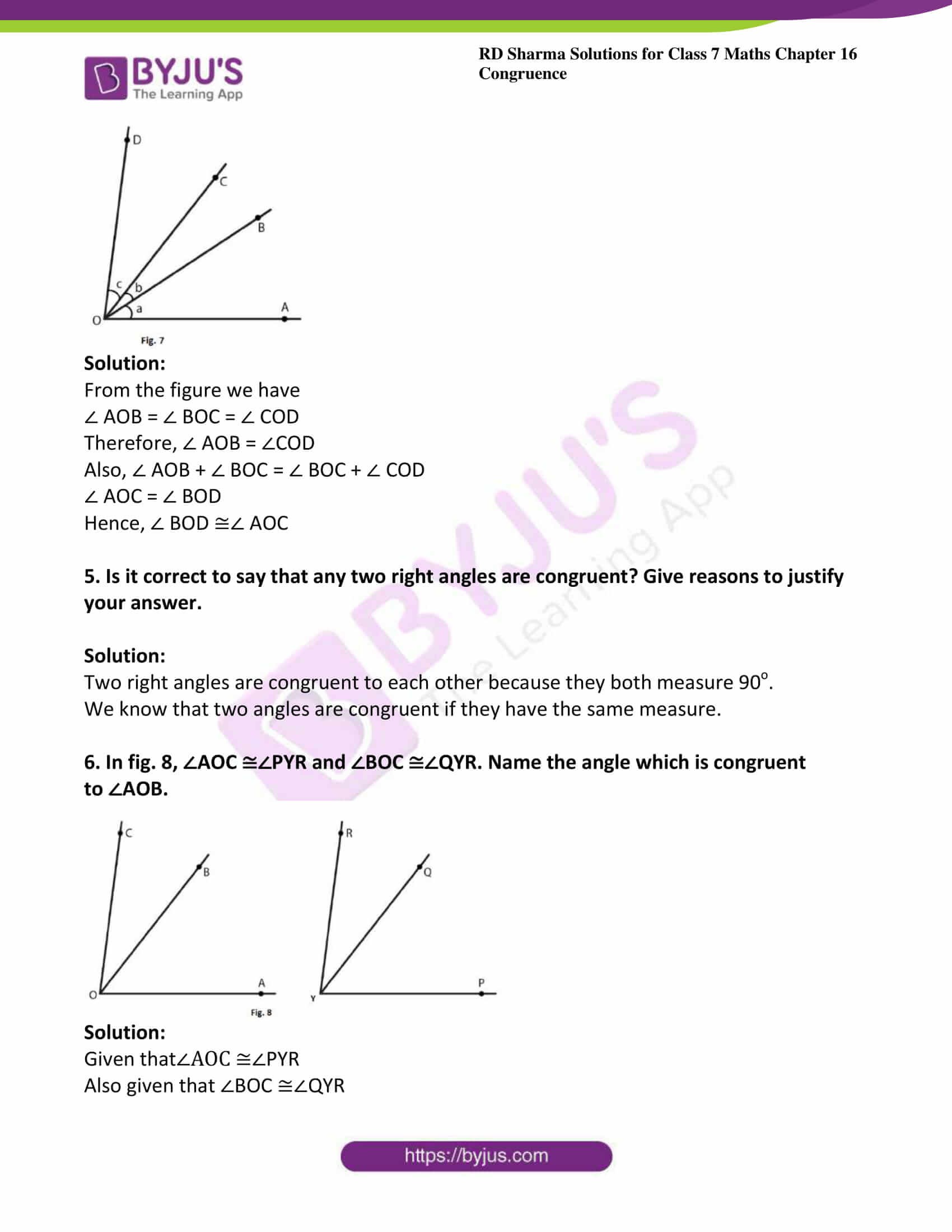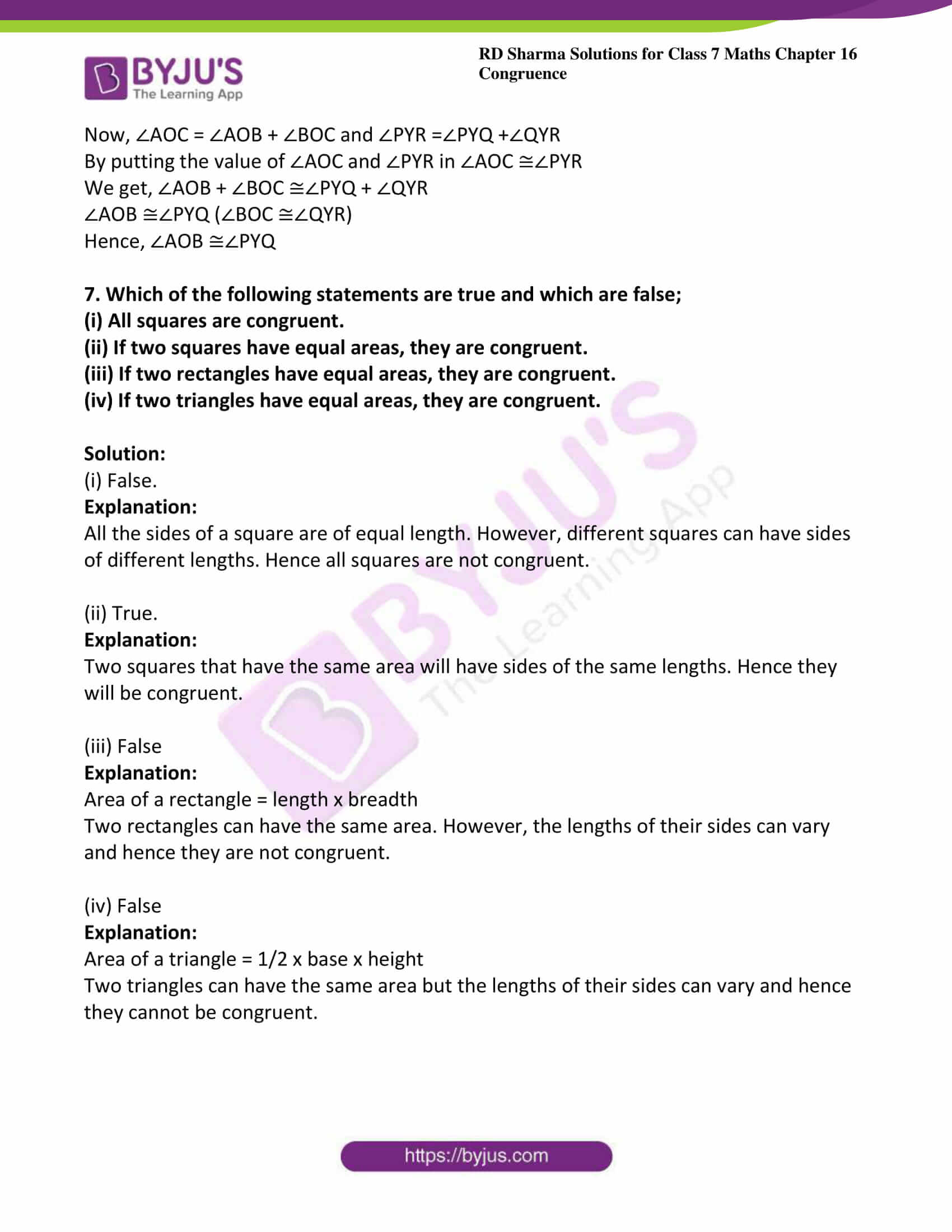### Access answers to Maths RD Sharma Solutions For Class 7 Chapter 16 – Congruence Exercise 16.1

Exercise 16.1 Page No: 16.3

1. Explain the concept of congruence of figures with the help of certain examples.

Solution:

Congruent objects or figures are exact copies of each other or we can say mirror images of each other. The relation of two objects being congruent is called congruence.

Consider Ball 1 and Ball 2. These two balls are congruent.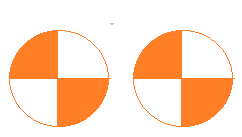Ball 1 Ball 2

Now consider the two stars below. Star A and Star B are exactly the same in size, colour and shape. These are congruent starsStar A Star B

2. Fill in the blanks:

(i) Two line segments are congruent if ……..

(ii) Two angles are congruent if ……..

(iii) Two square are congruent if ………

(iv) Two rectangles are congruent if ………

(v) Two circles are congruent if …….

Solution:

(i) They are of equal lengths

(ii) Their measures are the same or equal.

(iii) Their sides are equal or they have the same side length

(iv) Their dimensions are same that is lengths are equal and their breadths are also equal.

3. In Fig. 6, ∠POQ ≅∠ROS, can we say that ∠POR ≅∠QOS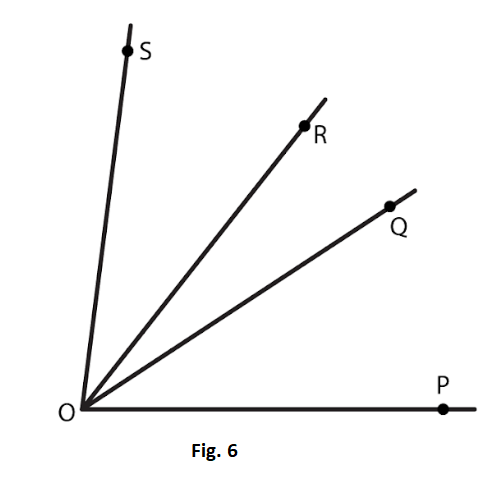Solution:

Given that

∠POQ ≅∠ROS

Also ∠ROQ ≅∠ROQ

Therefore adding ∠ROQ to both sides of ∠POQ ≅∠ROS,

We get, ∠POQ + ∠ROQ ≅∠ROQ + ∠ROS

Therefore, ∠POR ≅∠QOS

4. In fig. 7, a = b = c, name the angle which is congruent to ∠AOC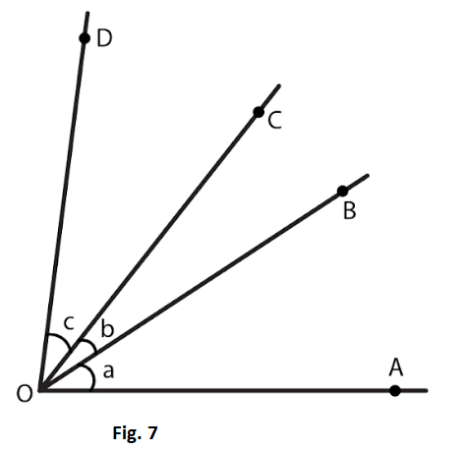Solution:

From the figure we have

∠ AOB = ∠ BOC = ∠ COD

Therefore, ∠ AOB = ∠COD

Also, ∠ AOB + ∠ BOC = ∠ BOC + ∠ COD

∠ AOC = ∠ BOD

Hence, ∠ BOD ≅∠ AOC

5. Is it correct to say that any two right angles are congruent? Give reasons to justify your answer.

Solution:

Two right angles are congruent to each other because they both measure 90o.

We know that two angles are congruent if they have the same measure.

6. In fig. 8, ∠AOC ≅∠PYR and ∠BOC ≅∠QYR. Name the angle which is congruent to ∠AOB.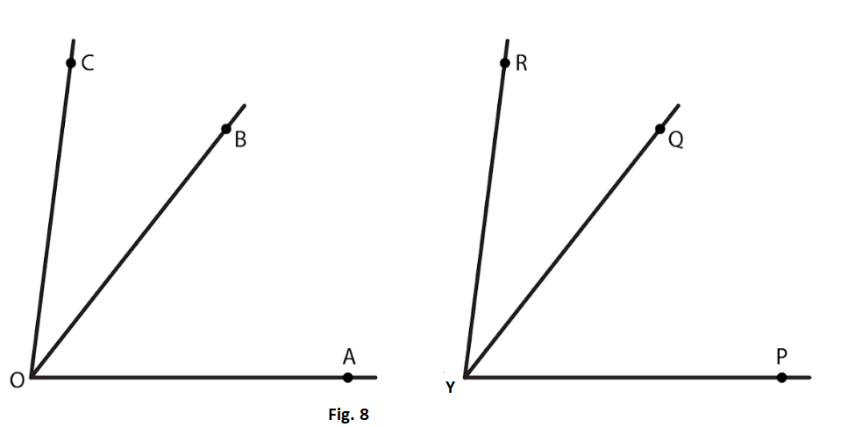Solution:

Given that∠AOC ≅∠PYR

Also given that ∠BOC ≅∠QYR

Now, ∠AOC = ∠AOB + ∠BOC and ∠PYR =∠PYQ +∠QYR

By putting the value of ∠AOC and ∠PYR in ∠AOC ≅∠PYR

We get, ∠AOB + ∠BOC ≅∠PYQ + ∠QYR

∠AOB ≅∠PYQ (∠BOC ≅∠QYR)

Hence, ∠AOB ≅∠PYQ

7. Which of the following statements are true and which are false;

(i) All squares are congruent.

(ii) If two squares have equal areas, they are congruent.

(iii) If two rectangles have equal areas, they are congruent.

(iv) If two triangles have equal areas, they are congruent.

Solution:

(i) False.

Explanation:

All the sides of a square are of equal length. However, different squares can have sides of different lengths. Hence all squares are not congruent.

(ii) True.

Explanation:

Two squares that have the same area will have sides of the same lengths. Hence they will be congruent.

(iii) False

Explanation:

Area of a rectangle = length x breadth

Two rectangles can have the same area. However, the lengths of their sides can vary and hence they are not congruent.

(iv) False

Explanation:

Area of a triangle = 1/2 x base x height

Two triangles can have the same area but the lengths of their sides can vary and hence they cannot be congruent.